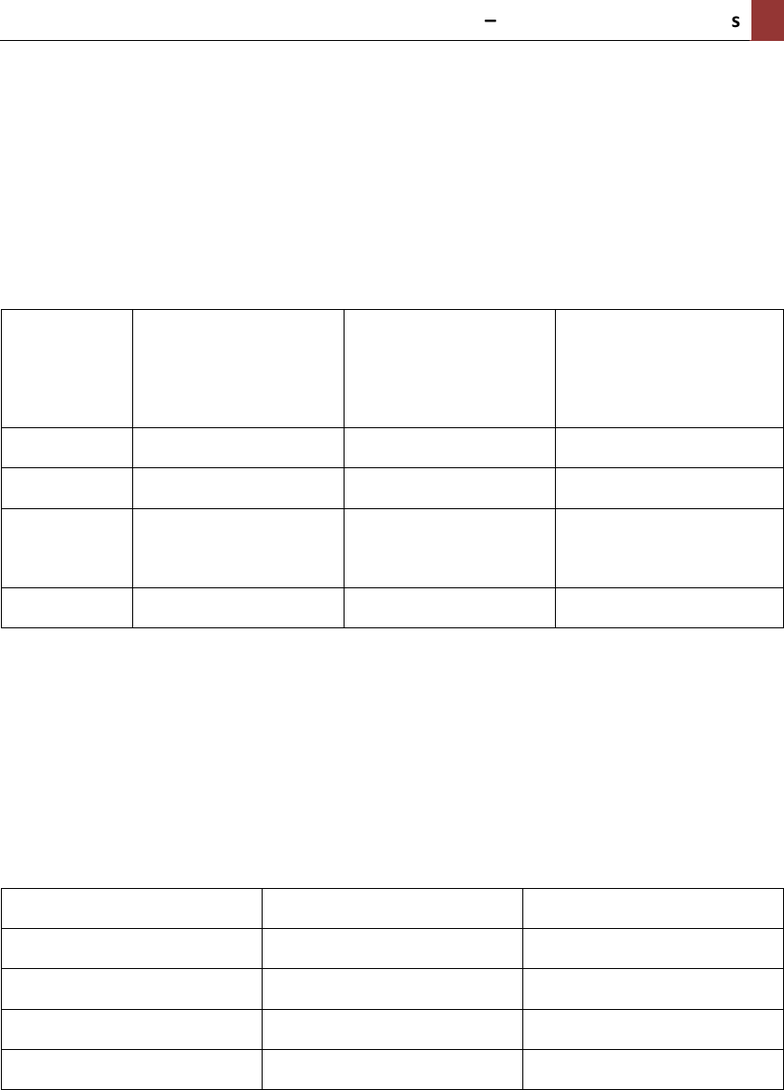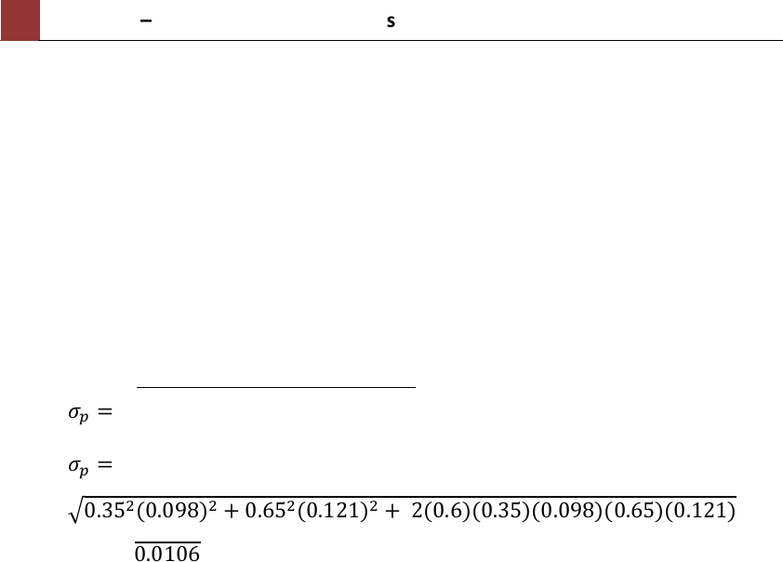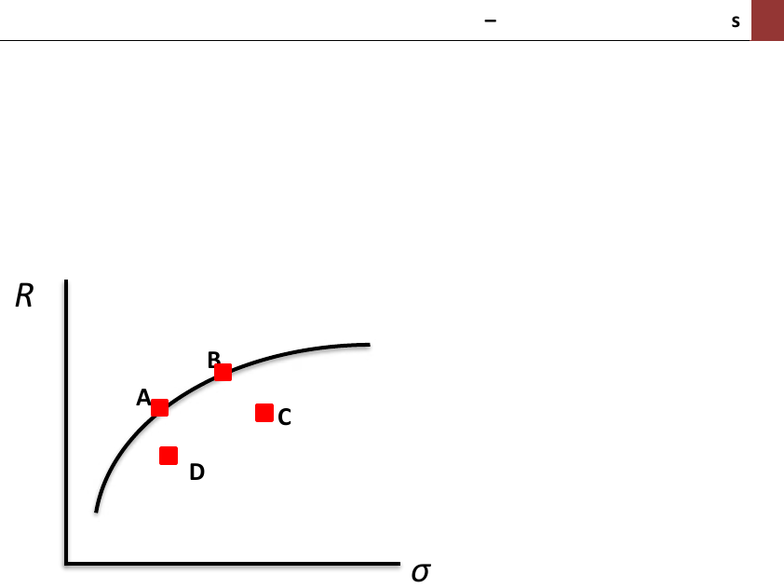# MMP321 Lecture 5: Topic 5 Practical Solutions

11 views9 pages
School
Course
Professor

For unlimited access to Study Guides, a Grade+ subscription is required.MMP321 Topic 5 Seminar Solutions
1
Topic 5: Portfolio theory and property securitization
Question 1:
Complete the following table for the four broad asset classes.
Asset class
Average return
(High, medium,
low etc.)
Liquidity
(High, medium,
low etc.)
Risk
(High, medium, low
etc.)
Cash
Very low
Very high
Very low
Bonds
Low
High
Low
Property
Moderate
Dependent on
structure*
Moderate
Shares
High
High
High
* Direct property investment is very illiquid, whilst securitized property
investment such as unlisted property trusts have higher liquidity. REITs have the
highest liquidity because they are listed on a securities exchange.
Question 2:
Using the information below, calculate the portfolio return.
Asset
Return
Weighting
A
12%
40%
B
9%
20%
C
15%
30%
D
13%
10%
SOLUTION:
Portfolio return = (12%)(0.4) + (9%)(0.2) + (15%)(0.3) + (13%)(0.1) = 12.4%
Question 3:
You have invested 35% of your wealth in asset X and 65% in asset Y. The average
return for X and Y is 10% and 14% respectively. The correlation coefficient is 0.6
Unlock document

This preview shows pages 1-3 of the document.
Unlock all 9 pages and 3 million more documents.

Already have an account? Log in2
MMP321 Topic 5 Seminar Solutions
and asset X has a standard deviation of 9.8%, while asset Y has a standard deviation
of 12.1%.
i) Calculate the expected return of the portfolio.
ii) Calculate the standard deviation of the portfolio.
SOLUTION:
Part i) Expected return on portfolio = (10%)(0.35) + (14%)(0.65) = 12.6%
Part ii) Portfolio standard deviation: (note: similar to calculating standard
deviation of a single asset, it is easier to input your values as decimals).

 
   
Question 4:
i) Download the file loaded in CloudDeakin titled “Topic 5 COR calculation
returns”. Using excel calculate the correlation coefficient for Brookfield
Prime Property Fund and Abacus Property Group.
ii) Why, when calculating the standard deviation of a portfolio, can’t we
simply estimate the weighted average of the standard deviation of the
individual assets?
SOLUTION:
Part i) In excel type (highlight cells) the following command:
CORREL(B2:B26,C2:C26)
Output should give you a correlation coefficient of 0.2492
Part ii) We need to consider the relationship between each asset. This is captured
by the correlation coefficient and is a measure of how two independent assets
move in relation to one another.
The correlation coefficient is bounded between -1 and +1.
-1 means the two assets move in opposite direction to each other. This results in
complete risk reduction.
+1 means the two assets move exactly the same and results in no risk reduction.
Unlock document

This preview shows pages 1-3 of the document.
Unlock all 9 pages and 3 million more documents.

Already have an account? Log inMMP321 Topic 5 Seminar Solutions
3
Question 5:
i) The diagram below displays the efficient frontier. Which portfolios are
efficient and which are inefficient?
ii) Why do we want our portfolios to sit on the efficient frontier?
SOLUTION:
Part i) Portfolios A and B are efficient because they sit on the efficient frontier
curve. Portfolio C and D are inefficient.
Part ii) Portfolios that sit on the efficient frontier provide us with the highest
possible return given a particular level of risk. Anything under the frontier gives
us a lower rate of return that we could achieve for the same level of risk if the
portfolio is on the efficient frontier.
Question 6:
Define the term ‘securitisation’ and give an example.
SOLUTION:
Securitisation is the issuing of securities backed by investment assets. An example
is when a property trusts purchases properties and sells units in the trust. The units
value is based on the market value of the underlying properties.
A
C
D
B
Unlock document

This preview shows pages 1-3 of the document.
Unlock all 9 pages and 3 million more documents.

Already have an account? Log in

# Get access

Grade+
\$10 USD/m
Billed \$120 USD annually
Homework Help
Class Notes
Textbook Notes
40 Verified Answers
Study Guides
1 Booster Class